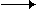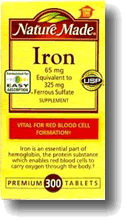Redox titrations

Reduction and oxidation reactions

Redox titrations depend on the transfer of electrons between the two reacting species in solution. They may or may not be self-indicating. Examples include:

• 1 Determination of copper(II) by displacement of iodine followed by titration with sodium thiosulfate.
• 2 Reaction of ethandioate ions with potassium manganate(VII).
• 3 Determination of iron(II) by reaction with potassium manganate(VII).

Potassium manganate(VII), KMnO4, is a self-indicating reagent, which turns from the deep purple manganate(VII) ions to the nearly colourless manganese(II) ions.

MnO4-(aq) + 8H+(aq) + 5eMn2+(aq) + 4H2O(l)

It is a good oxidising agent in acidic solution.top

Determination of iron(II) by manganate(VII) in acidic solution

Iron(II) ions are pale green in solution. They are oxidised by manganate(VII) ions in acidic solution to iron(III), which are pale yellow in solution.

MnO4-(aq) + 8H+(aq) + 5Fe2+(aq)Mn2+(aq) + 4H2O(l) + 5Fe3+(aq)

The stoichiometric ratio of reaction is given by the coefficients of the balanced equation above. If 25ml of a known concentration of potassium manganate(VII) solution is placed in a conical flask and an unknown iron(II) solution run into the flask from a burette, the end point of the titration is given by the disappearance of the purple manganate(VII) ion colour.

This reaction scheme may be used to determine several things. For example:

• 1 The concentration of an unknown iron(II) solution
• 2 The number of moles of water of crystallisation in an iron(II) salt.
• 3 The percentage iron in an alloy
• 4 The mass of iron in an iron tablet

The mass of iron in an iron tablet

BackgroundIron supplements contain soluble iron(II) salts to replenish them in the human body in case of deficiency. Iron is an essential mineral used in many functions, specifically in the transport of oxygen. Iron deficiency can occur through poor diet, or as a consequence of blood loss through menstruation or injury.

Iron supplements usually contain about 50mg per tablet of iron(II).

Potassium manganate(VII), Mr = 158.034, is a good primary standard, being available to a high degree of purity, having a large relative mass and being stable in the environment.

Experimental

Weigh out a iron tablet in a weighing boat and transfer to a beaker.

Add 100 ml of deionised water and heat to dissolve the tablet.

Allow the solution to cool and transfer to a 250 ml volumetric flask and make up to the mark with deionised water.

Prepare 1000 ml of 0.01 mol dm-3 potassium manganate(VII) solution by accurately weighing out approximately 0.01 moles of potassium manganate(VII) and dissolving in 100 ml of 2 mol dm-3 sulphuric acid. This is transferred to a 1000 ml volumetric flask and made up to the mark with deionised water.

Pipette a 25 ml aliquot of the potassium manganate(VII) solution into a conical flask

Run the unknown iron(II) solution into the flask from a burette, recording the volume when the purple colour of the manganate(VII) ions has just decolourised.

Repeat the titration until concordant results are obtained.

Data recording

Results from a typical experiment are shown below:

• Mass of potassium manganate(VII) used: 1.58 g
• Concentration of standard potassium manganate(VII) solution = 1.58/158.034 = 0.01 mol dm-3
• Volume of potassium manganate(VII) used for the titration = 10ml.

Titration results:

 titre initial/ml ± 0.05 final/ml ± 0.05 volume/ml ± 0.1 1 0.00 28.20 28.20 2 0.05 27.95 27.90 3 0.00 27.90 27.90 average of concordant titres 27.90

Data processing

Moles of manganate(VII) = 0.01 x 0.01 = 0.0001 mol

Therefore, from the reaction stoichiometry, moles of iron(II) = 5 x 0.0001 = 5.00 x 10-4 mol

This is in 27.90 ml, therefore in 250 ml there is (250/27.9) x 5.00 x 10-4 = 8.96 x 5 x 10-4 moles of iron = 4.48 x 10-3 mol

Therefore mass of iron in 250 ml = 56 x 4.48 x 10-3 g = 0.25 g

Hence, mass of iron per tablet = 250 mgtop

A2 Inorganic Chemistry experiments

A2 Physical Chemistry experiments

A2 Organic Chemistry experiments

 Task Possible context Prepare a solid organic compound The preparation of aspirin Purify an organic solid The recrystallisation of impure benzenecarboxylic acid from hot water Test the purity of an organic solid Determine the melting point of benzenecarboxylic acidtop